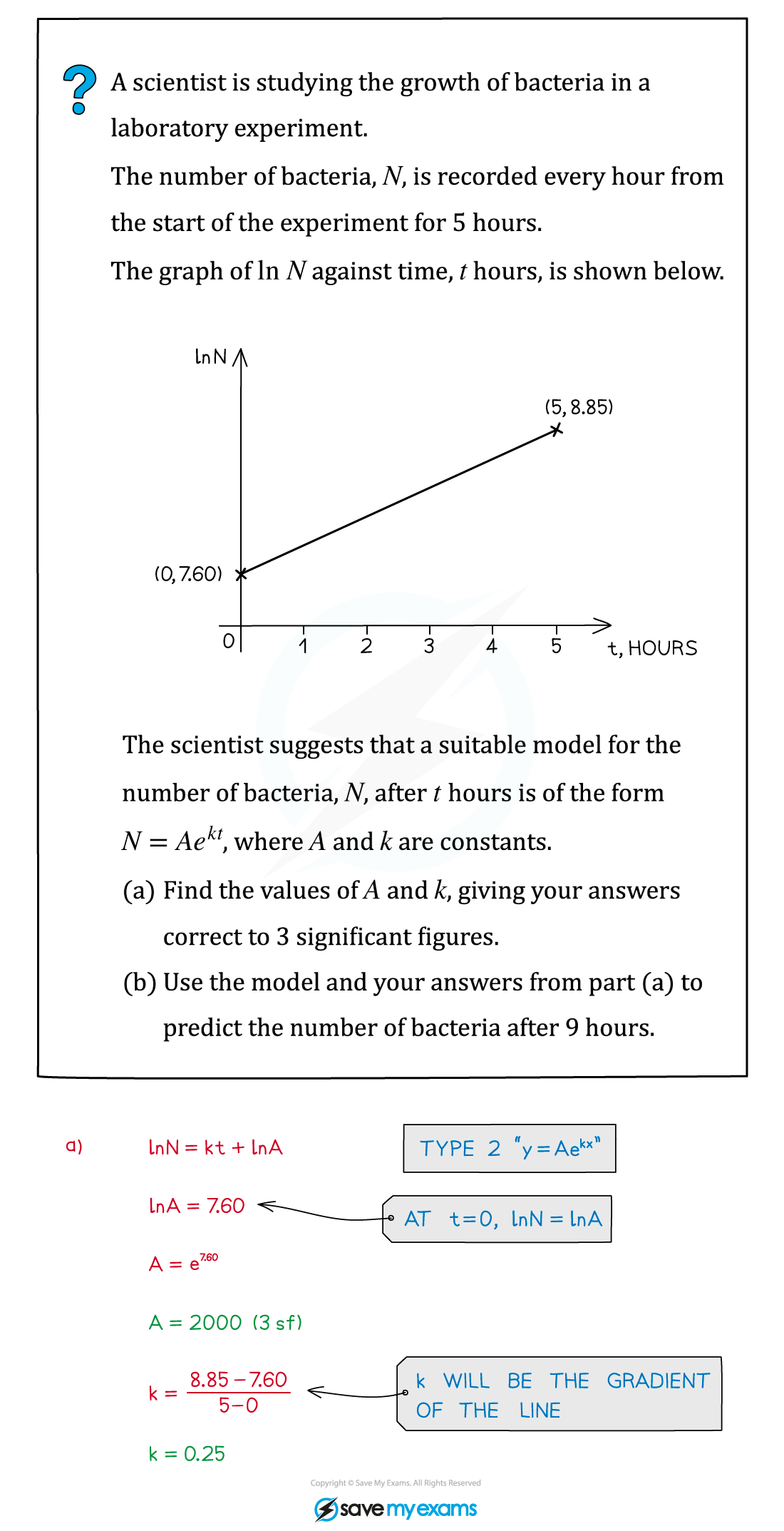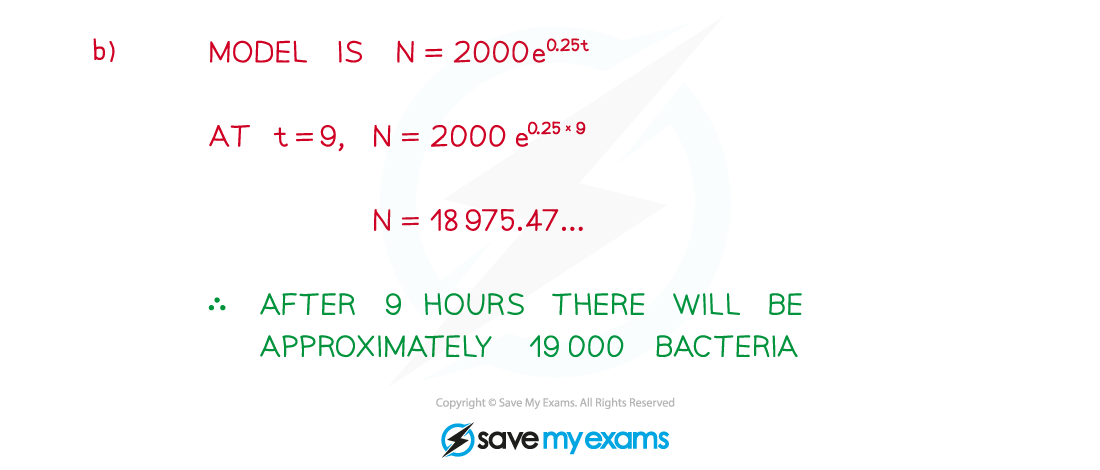# AQA A Level Maths: Pure复习笔记6.3.3 Using Log Graphs in Modelling

### Using Log Graphs in Modelling

#### What are log graphs?

• Log graphs are used when the scale of a graph increases or decreases exponentially
• It can very difficult to read specific values from graphs with these scales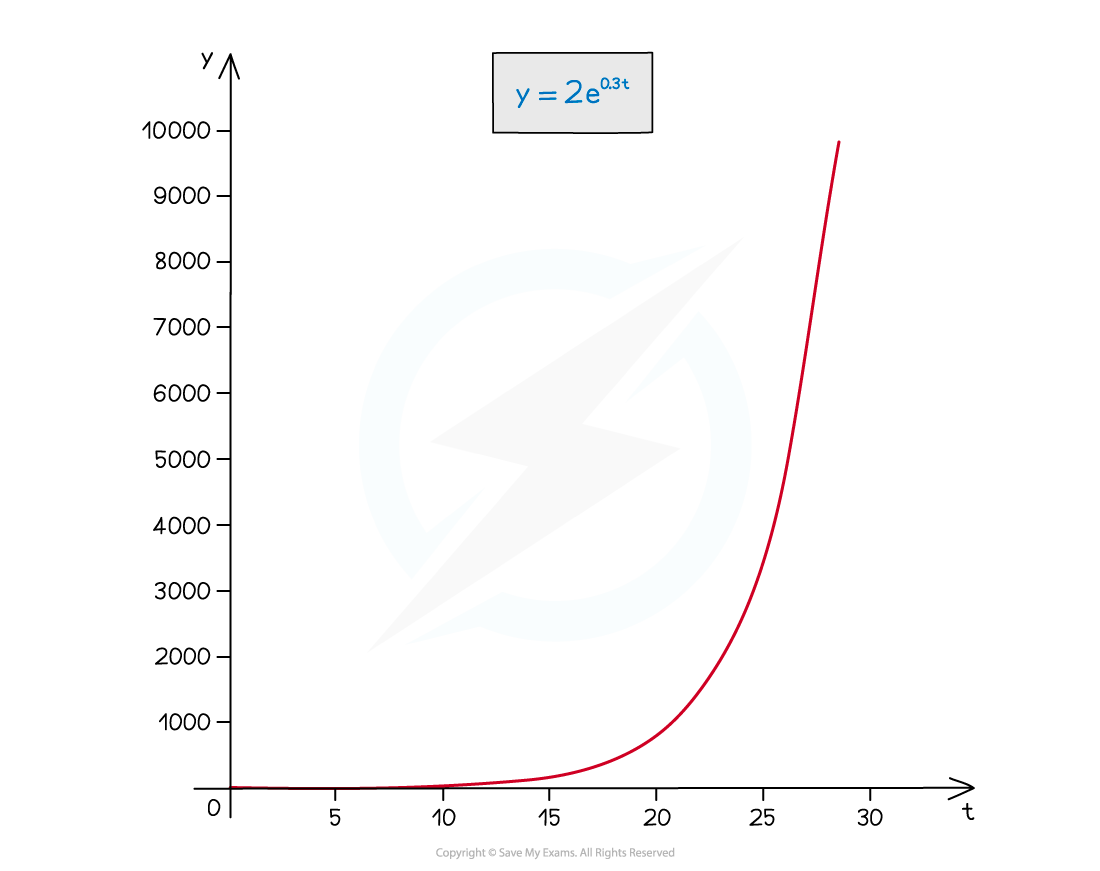• Taking ln of both sides allows the equation to be rearranged into the form “y = mx + c”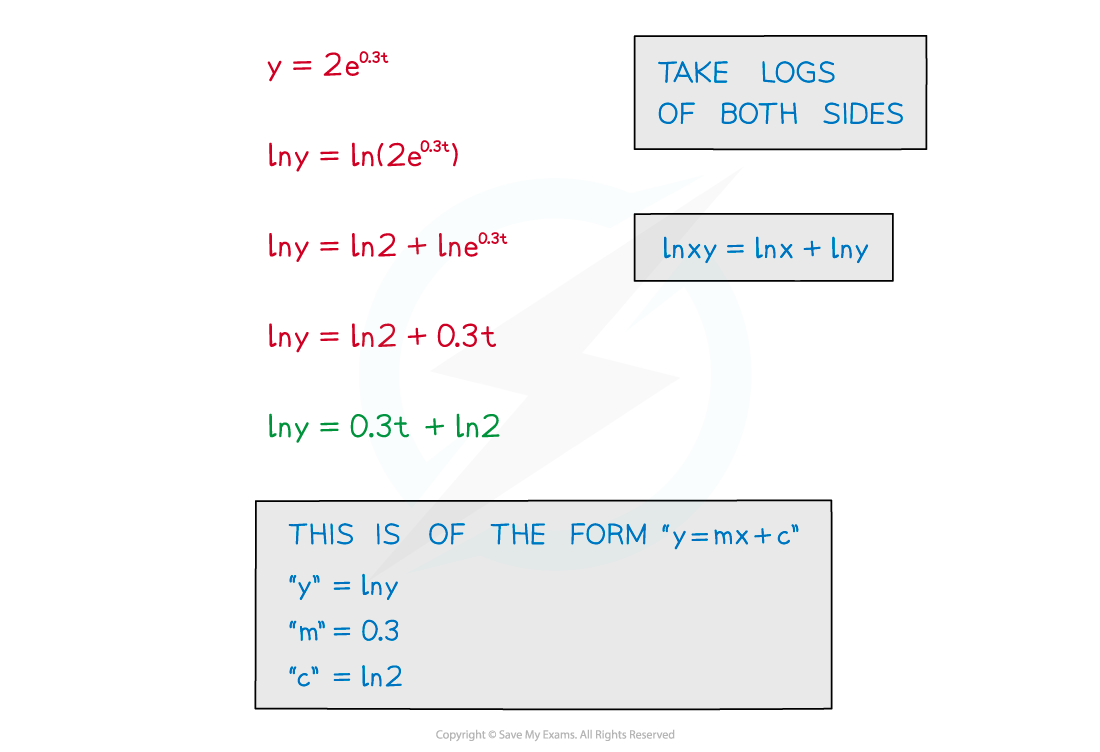• Plotting ln y against t produces a straight line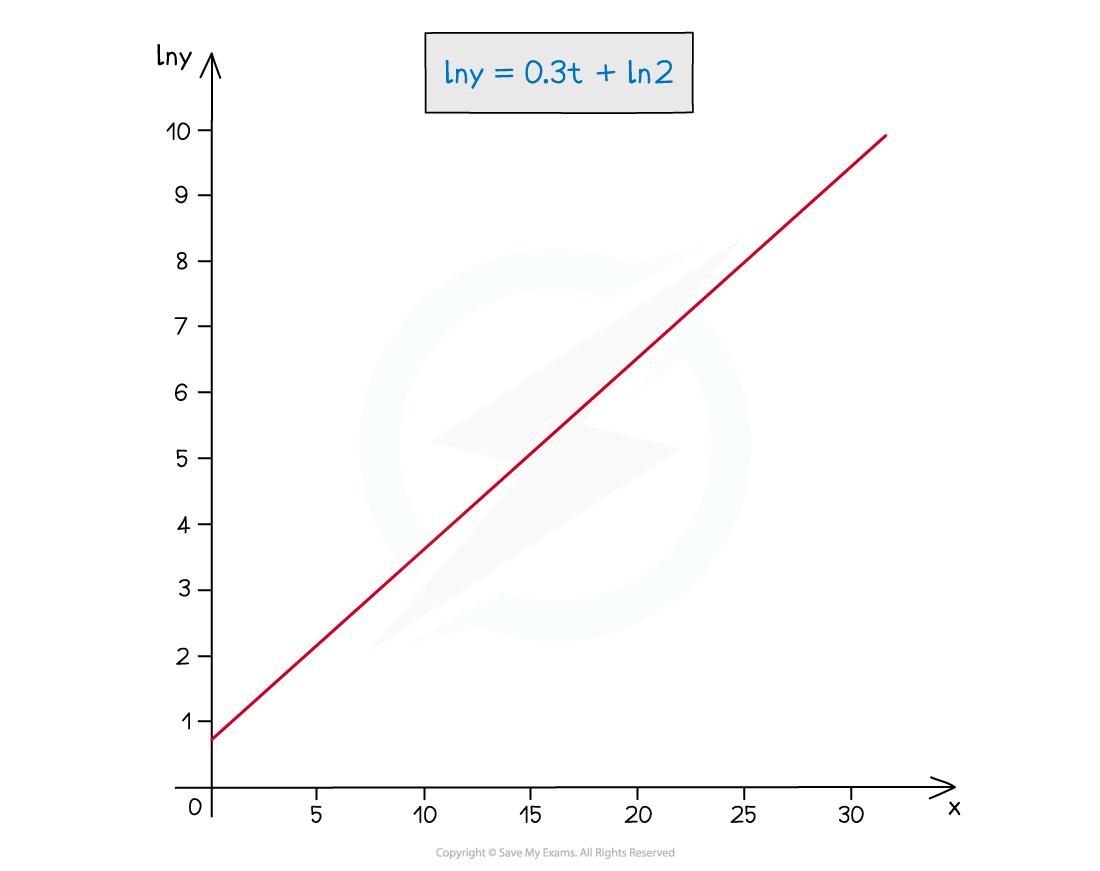• Note the second graph has ln y on the y-axis
• Log graphs have at least one logarithmic axis
• Reading a value for ln y at t = 20 is easier than reading the value for y
• Logarithmic axes are used where a wide range of numbers can occur
• it makes numbers smaller and easier to deal with
• a curve can be turned into a straight line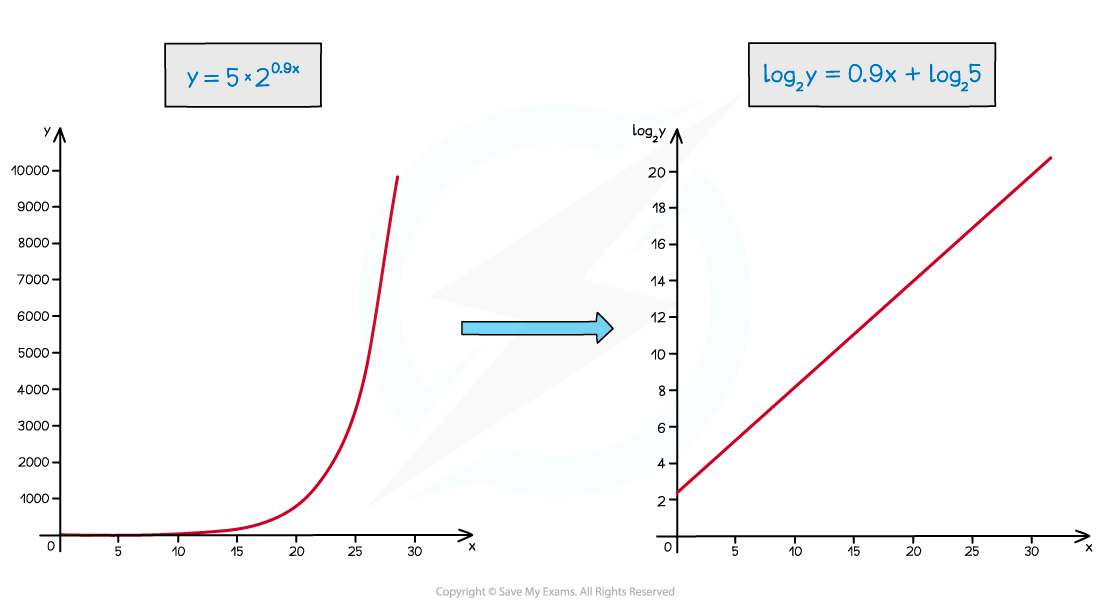#### How do I use a logarithmic graph with exponential modelling?

• Exponential models are of the form
• y=Abkx (growth)
• y=Ab-kx (decay)
• To use a model to make predictions the values of A, b and k are needed
• A, b and k will usually be estimated from observed data values
• A may be known exactly, as it is a starting/initial value
• Estimating from a straight line graph is easier than from a curve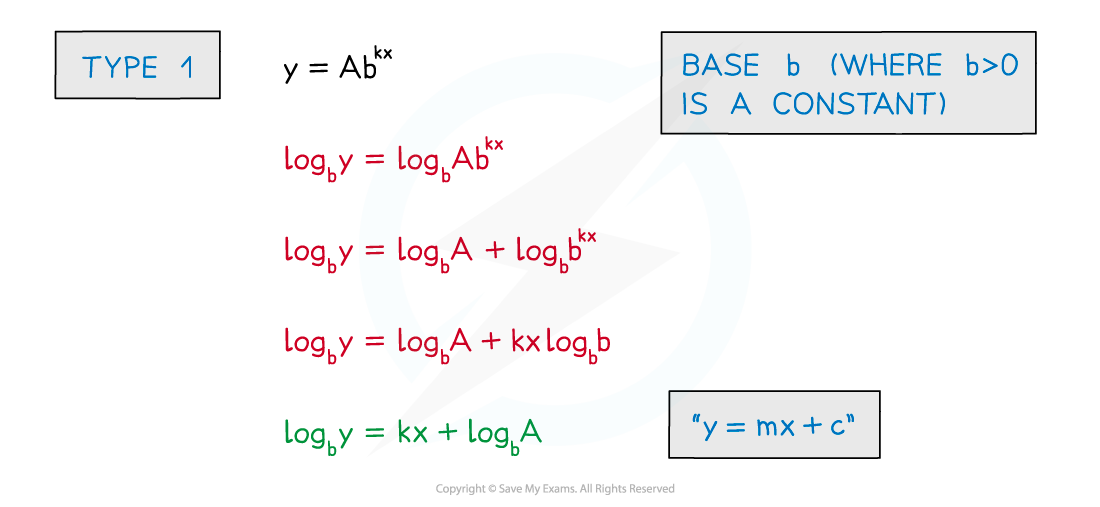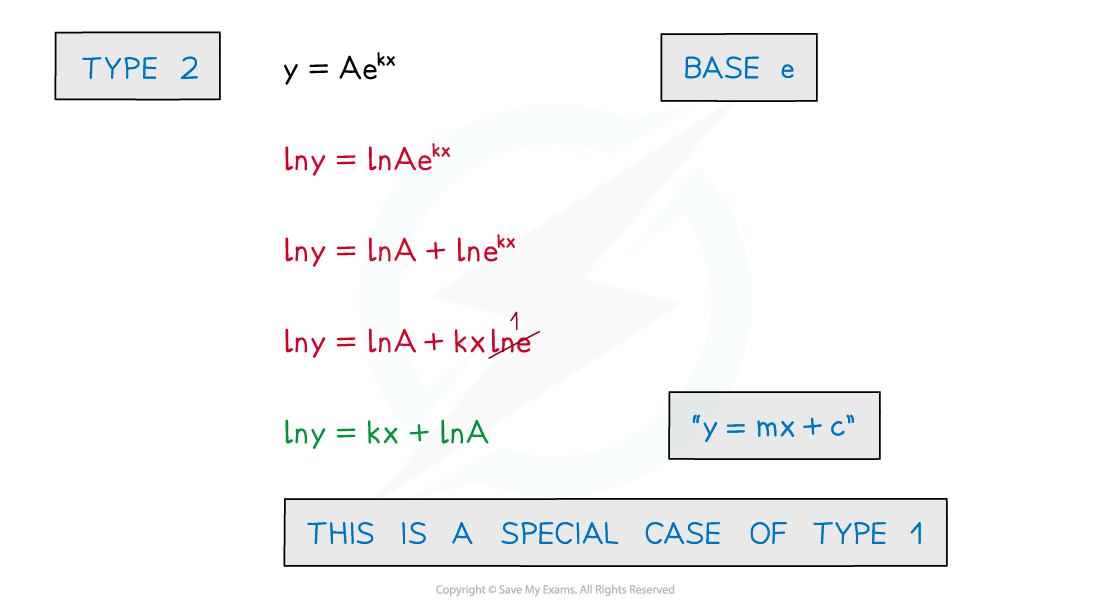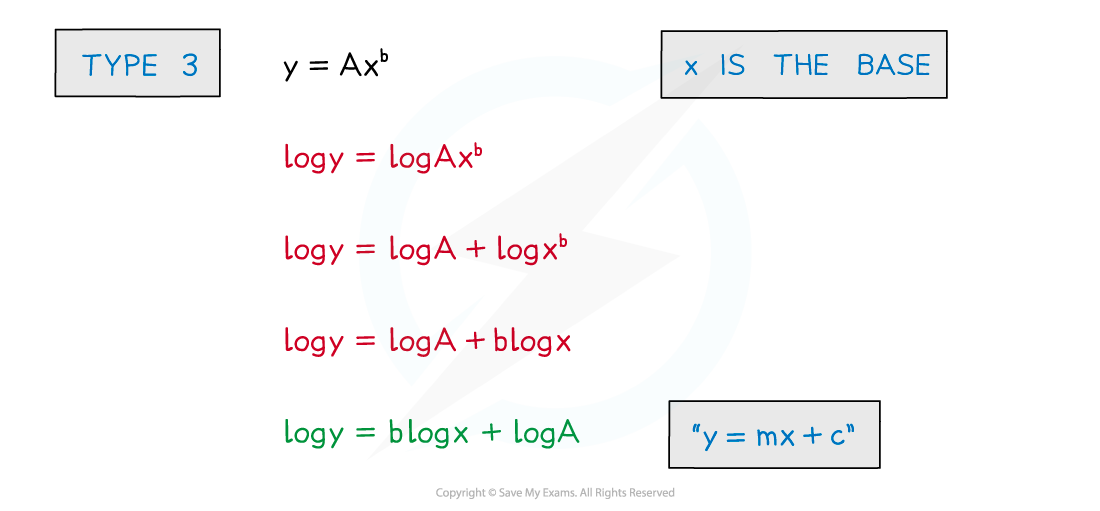#### Worked Example